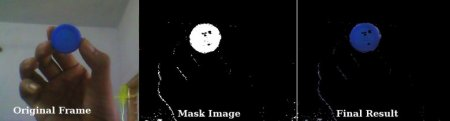OpenCV  4.4.0 Open Source Computer Vision
Changing Colorspaces

## Goal

• In this tutorial, you will learn how to convert images from one color-space to another, like BGR $$\leftrightarrow$$ Gray, BGR $$\leftrightarrow$$ HSV, etc.
• In addition to that, we will create an application to extract a colored object in a video
• You will learn the following functions: cv.cvtColor(), cv.inRange(), etc.

## Changing Color-space

There are more than 150 color-space conversion methods available in OpenCV. But we will look into only two, which are most widely used ones: BGR $$\leftrightarrow$$ Gray and BGR $$\leftrightarrow$$ HSV.

For color conversion, we use the function cv.cvtColor(input_image, flag) where flag determines the type of conversion.

For BGR $$\rightarrow$$ Gray conversion, we use the flag cv.COLOR_BGR2GRAY. Similarly for BGR $$\rightarrow$$ HSV, we use the flag cv.COLOR_BGR2HSV. To get other flags, just run following commands in your Python terminal:

>>> import cv2 as cv
>>> flags = [i for i in dir(cv) if i.startswith('COLOR_')]
>>> print( flags )
Note
For HSV, hue range is [0,179], saturation range is [0,255], and value range is [0,255]. Different software use different scales. So if you are comparing OpenCV values with them, you need to normalize these ranges.

## Object Tracking

Now that we know how to convert a BGR image to HSV, we can use this to extract a colored object. In HSV, it is easier to represent a color than in BGR color-space. In our application, we will try to extract a blue colored object. So here is the method:

• Take each frame of the video
• Convert from BGR to HSV color-space
• We threshold the HSV image for a range of blue color
• Now extract the blue object alone, we can do whatever we want on that image.

Below is the code which is commented in detail:

import cv2 as cv
import numpy as np
while(1):
# Take each frame
# Convert BGR to HSV
hsv = cv.cvtColor(frame, cv.COLOR_BGR2HSV)
# define range of blue color in HSV
lower_blue = np.array([110,50,50])
upper_blue = np.array([130,255,255])
# Threshold the HSV image to get only blue colors
# Bitwise-AND mask and original image
cv.imshow('frame',frame)
cv.imshow('res',res)
k = cv.waitKey(5) & 0xFF
if k == 27:
break

Below image shows tracking of the blue object:image
Note
There is some noise in the image. We will see how to remove it in later chapters.
This is the simplest method in object tracking. Once you learn functions of contours, you can do plenty of things like find the centroid of an object and use it to track the object, draw diagrams just by moving your hand in front of a camera, and other fun stuff.

## How to find HSV values to track?

This is a common question found in stackoverflow.com. It is very simple and you can use the same function, cv.cvtColor(). Instead of passing an image, you just pass the BGR values you want. For example, to find the HSV value of Green, try the following commands in a Python terminal:

>>> green = np.uint8([[[0,255,0 ]]])
>>> hsv_green = cv.cvtColor(green,cv.COLOR_BGR2HSV)
>>> print( hsv_green )
[[[ 60 255 255]]]

Now you take [H-10, 100,100] and [H+10, 255, 255] as the lower bound and upper bound respectively. Apart from this method, you can use any image editing tools like GIMP or any online converters to find these values, but don't forget to adjust the HSV ranges.